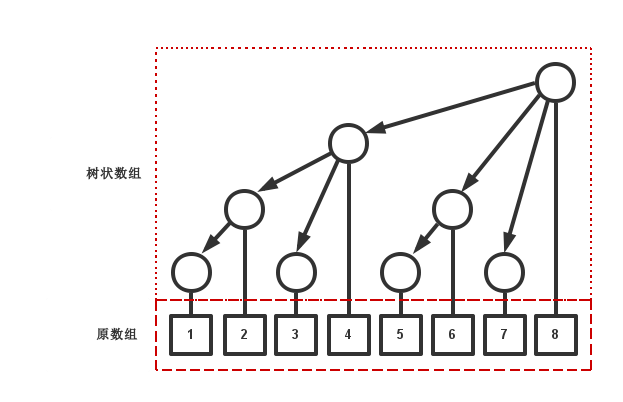# 树状数组

## 点修改区间查询

• 修改某一位置上的数
• 查询某一位置前缀和1 2 function LOWBIT(x): return x & (-x) 

  1 2 3 4 5 6 7 8 9 10 11 12 13 14 15 16 17 18 // 修改单点 function MODIFY(x, delta): if x < 1: return while x <= n: fenwick[x] += delta x += LOWBIT(x) // 查询前缀和 function QUERY(right): answer = 0 while right > 0: answer += fenwick[right] right -= LOWBIT(right) return answer 

## 区间修改点查询

• 修改一段前缀和
• 查询某一个位置上数的大小1 2 3 4 5 6 7 8 9 10 11 12 13 14 15 16 17 18 // 修改 function MODIFY(right, delta): while right > 0: fenwick[right] += delta right -= LOWBIT(right) // 查询 function QUERY(x): if x < 1: return 0 answer = 0 while x <= n: answer += fenwick[x] x += LOWBIT(x) return answer 

## 区间修改区间查询

• 修改一段前缀和
• 查询一段前缀和

  1 2 3 4 5 6 7 8 9 10 11 12 13 14 15 16 17 18 19 20 21 22 23 24 25 26 27 28 29 30 31 32 // 修改 function MODIFY(right, delta): if right < 1: return i = right while i > 0: fenwick1[i] += delta i -= LOWBIT(i) i = right while i <= n: fenwick2[i] += delta * right // 注意是区间每一个数都加上了delta i += LOWBIT(i) function QUERY(right): if right < 1: return 0 answer = 0 i = right while i <= n: // 统计父亲上的信息 answer += fenwick1[i] i += LOWBIT(i) answer *= right // 因为是每个数所加上的数，所以要乘以个数 i = right - 1 while i > 0: // 统计儿子信息 answer += fenwick2[i] i -= LOWBIT(i) return answer 

## 优势与缺点

• 代码短小，常数小
• 易于调试
• 容易扩展到高维度的数据

• 只能用于求和3，不能求最大/小值
• 不能动态插入，面多多维数据时空间压力大
• 不能可持久化

1. 如果下标一定要为$0$，可以考虑将其全部加$1$。

2. 当然也可以直接替代原数组。

3. 或者说具有区间减法性质的数据。